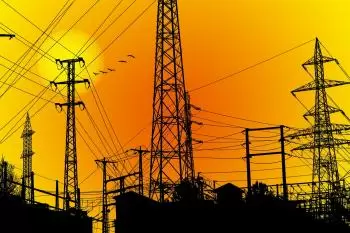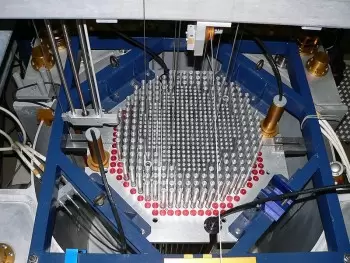# Different Forms of Energy and Examples, Types of EnergyThere are different ways to classify the different types of energy. The main types are the following:

• Nuclear energy: is obtained from the energy contained within the nuclei of atoms.

• Mechanical energy includes two types of energy: potential and kinetic. It depends on the position and speed of a body.

• Thermodynamics refers to the internal energy of a thermodynamic system.

• Electromagnetic energy is generated through electric and magnetic fields such as radiant energy and light energy.

• Electrical energy is related to the movement of electrons in an electrical conductor.

• Magnetic energy is the energy that is generated in a magnetic field.

• Sound energy is related to sound and its propagation by waves.

• Chemical energy is stored in the bonds of chemical compounds.

According to the law of conservation of energy, the different forms of energy can be converted to other forms of energy. However, in any case, they can not be created or destroyed.

Depending on the origin of the energy sources, we distinguish the following types:

• Renewable energies come from unlimited energy sources.

• Non-renewable energies come from exhaustible sources such as fossil fuels.

Below we define the most important types of energy:

Nuclear energy

Nuclear energy is the chemical energy that holds the sub-particles of an atom's nucleus together: the protons and the neutrons.The mass of an atomic nucleus is less than the sum of the masses of the elementary particles that form it (mass defect). This mass defect is due to the appearance of cohesion energy absorbed by the nuclear structure, which can be calculated using Einstein's theory of relativity.

The forces that hold atomic nuclei together possess a large amount of energy. Nuclear reactors are capable of releasing this energy through a chemical reaction.

Some examples of atomic energy are nuclear power plants, atomic bombs, and radiology devices.

## Mechanical Energy

Mechanical energy is due to the geometric and dynamic variables of the system, from a macroscopic point of view, and is the one that responds to the most straightforward mathematical scheme.

There are two types of mechanical energy:

• Kinetic energy is the energy coming from motion energy.

• Potential energy is associated with the position of an item.

The definition of mechanical energy in a material body is the sum of its kinetic and potential energies. Kinetic energy is proportional to the speed of the body. The potential energy depends on the body's position in the force field surrounding it. If this force is gravity, it is called gravitational energy.

Some examples of mechanical energy are the car's speed car (kinetic) and the water at the highest point of a waterfall. In a hydropower plant, water has kinetic energy and potential energy.

## Thermodynamic Energy

Thermodynamic energy is energy in the form of heat. It makes sense from a molecular point of view.

The internal energy U of a system is the sum of the kinetic energies of the molecules that compose it and the potential energy of the forces between them.

Each of the atoms or molecules of a body is constantly in motion. This motion can be rotation, translation, or vibration to the equilibrium position. Finally, its intensity depends more or less on the temperature.

From this point of view, we can say that thermal energy is equivalent to the kinetic energy of molecules, as formulated by L. Boltzmann in his kinetic theory of gases. We speak about heat energy when this energy is released or absorbed.

Internal energy is not a value that can be measured. We can only measure variations in energy between two states of the system.

Examples of this kind of energy are the heat energy generated by a kettle, a hot air balloon, and a thermal power plant.

## Electric Power

Electrical energy is energy that is transmitted as electrical work by an electrical current in a circuit. As a concept, it can refer to several closely related forms of energy: the energy in the electric field, the potential energy of electric charge, and electric current energy.

Some examples of electric power are PV solar energy, an electric generator, and a high-voltage network.

Author:

Published: May 14, 2020
Last review: July 4, 2022